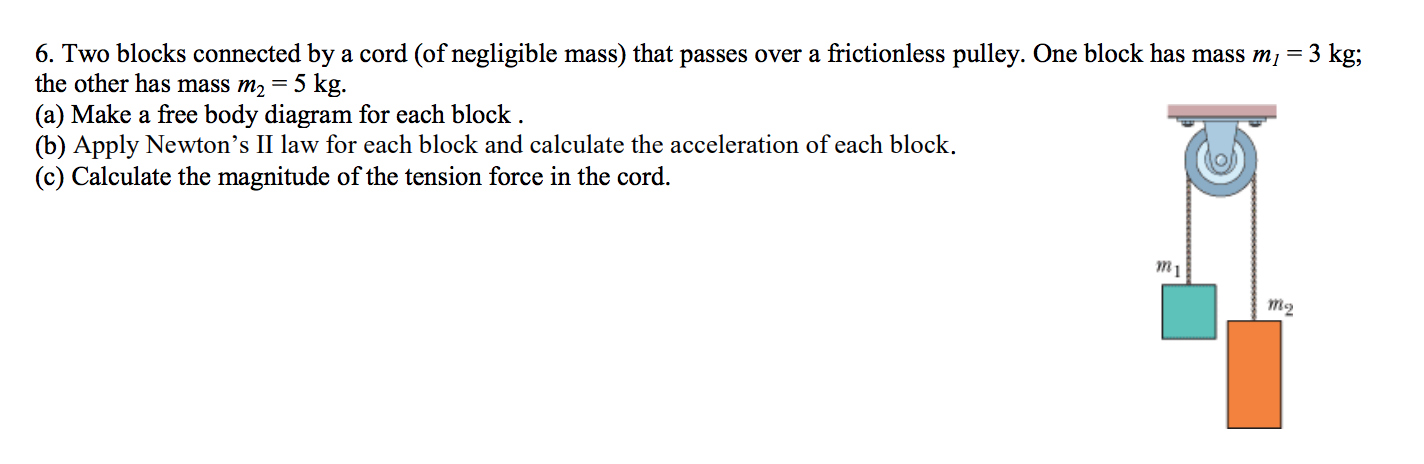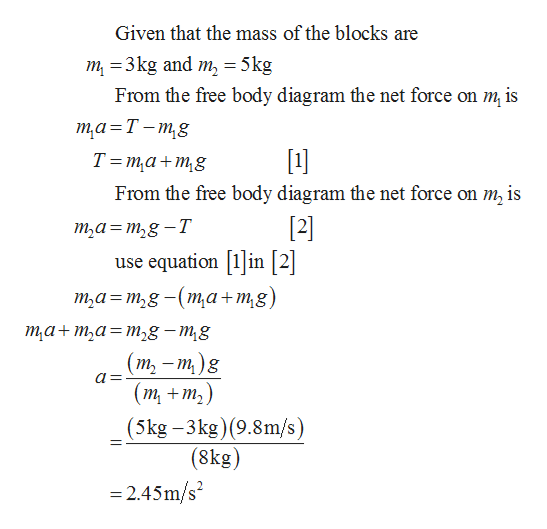# 6. Two blocks connected by a cord (of negligible mass) that passes over a frictionless pulley. One block has mass mi = 3 kg;the other has mass m2 = 5 kg.(a) Make a free body diagram for each block .(b) Apply Newton's II law for each block and calculate the acceleration of each block.(c) Calculate the magnitude of the tension force in the cord.

Question
1 viewshelp_outlineImage Transcriptionclose6. Two blocks connected by a cord (of negligible mass) that passes over a frictionless pulley. One block has mass mi = 3 kg; the other has mass m2 = 5 kg. (a) Make a free body diagram for each block . (b) Apply Newton's II law for each block and calculate the acceleration of each block. (c) Calculate the magnitude of the tension force in the cord. fullscreen
check_circle

Step 1

(a)  The free body diagram of the system is given below

Step 2

(b) Write the expression for accelera...help_outlineImage TranscriptioncloseGiven that the mass of the blocks are m 3kg and m 5kg From the free body diagram the net force on m is та-Т-тg T ma+mg From the free body diagram the net force on m2 is  use equation 1in 2 т,а%3Dт,g -T т,а%3 т,g - (та+тg) та+m,а%3Dт,g — mg (т, — т)g a= (т, +т,) (5kg-3kg)(9.8m/s) (8kg) 2.45m/s2 fullscreen

### Want to see the full answer?

See Solution

#### Want to see this answer and more?

Solutions are written by subject experts who are available 24/7. Questions are typically answered within 1 hour.*

See Solution
*Response times may vary by subject and question.
Tagged in

### Newtons Laws of Motion